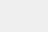# 使用Python+opencv进行图像处理(一) | 视觉入门1. 理解颜色模型与在图像上绘制图形（图像处理基本操作）。

2. 基本的图像处理与过滤。

3. 从特征检测到人脸检测（TBU

OpenCVOpen Source Computer Vision的缩写，由英特尔公司于1999年推出。它最初是用C/ C++编写的，所以你可能会看到更多用C语言而不是Python编写的教程。但现在它在Python中也被广泛用于计算机视觉。首先，让我们为使用OpenCV配置环境。安装过程如下，详细安装描述参看https://pypi.org/project/opencv-python/

``pip install opencv-python==3.4.2pip install opencv-contrib-python==3.3.1``

``import cv2cv2.__version__``

``import numpy as npimport matplotlib.pyplot as plt%matplotlib inline# Import the imageimg = cv2.imread('burano.jpg')plt.imshow(img)````# Convert the image into RGBimg_rgb = cv2.cvtColor(img, cv2.COLOR_BGR2RGB)plt.imshow(img_rgb)````# Convert the image into gray scaleimg_gray = cv2.cvtColor(img, cv2.COLOR_BGR2GRAY)plt.imshow(img_gray, cmap = 'gray')``

``# Plot the three channels of the imagefig, axs = plt.subplots(nrows = 1, ncols = 3,figsize = (20, 20))for i in range(0, 3):    ax =axs[i]   ax.imshow(img_rgb[:, :, i], cmap = 'gray')plt.show()``HSVHLS有一些不同。正如在上图看到的那样，他们有一个三维的表达，更类似于人类的感知方式。HSV代表色调、饱和度和色值。HSL代表色调、饱和度和亮度。HSV的中轴是色值，HSL的中轴是光量。沿着中心轴的角度，有色调和实际的颜色。与中心轴的距离属于饱和度。转换颜色模型的方法如下。

``# Transform the image into HSV and HLS modelsimg_hsv = cv2.cvtColor(img, cv2.COLOR_BGR2HSV)img_hls = cv2.cvtColor(img, cv2.COLOR_BGR2HLS)# Plot the converted imagesfig, (ax1, ax2) = plt.subplots(nrows = 1, ncols =2, figsize = (20, 20))ax1.imshow(img_hsv)ax2.imshow(img_hls)plt.show()``(https://towardsdatascience.com/finding-lane-lines-simple-pipeline-for-lane-detection-d02b62e7572b``# Copy the imageimg_copy = img.copy()# Draw a rectanglecv2.rectangle(img_copy, pt1 = (800, 470), pt2 =(980, 530),             color = (255, 0, 0), thickness = 5)plt.imshow(img_copy)````# Draw a circlecv2.circle(img_copy, center = (950, 50), radius =50,          color = (0, 0, 255), thickness = 5)plt.imshow(img_copy)，````# Add textcv2.putText(img_copy, text = "the Wall ofLove",           org = (250, 250),           fontFace = cv2.FONT_HERSHEY_DUPLEX,           fontScale = 2,           color = (0, 255, 0),           thickness = 2,           lineType = cv2.LINE_AA)plt.imshow(img_copy)``

``# Step 1. Define callback functiondef draw_circle(event, x, y, flags, param):    ifevent == cv2.EVENT_LBUTTONDOWN:           cv2.circle(img, center = (x, y), radius = 5,                       color = (87, 184, 237), thickness = -1)    elifevent == cv2.EVENT_RBUTTONDOWN:                  cv2.circle(img, center = (x, y), radius = 10,                        color = (87, 184, 237),thickness = 1)``

cv2.EVENT_RBUTTONDOWN记录位置数据。把鼠标的位置设置为圆心(x, y)，并绘制圆圈。

``# Step 2. Call the windowimg = cv2.imread('map.png')cv2.namedWindow(winname = 'my_drawing')cv2.setMouseCallback('my_drawing', draw_circle)``

``# Step 3. Executionwhile True:   cv2.imshow('my_drawing',img)    ifcv2.waitKey(10) & 0xFF == 27:       breakcv2.destroyAllWindows()````# Initializationdrawing = Falseix = -1iy = -1# create a drawing functiondef draw_rectangle(event, x, y, flags, params):    globalix, iy, drawing    ifevent == cv2.EVENT_LBUTTONDOWN:       drawing = True        ix,iy = x, y    elifevent == cv2.EVENT_MOUSEMOVE:        ifdrawing == True:           cv2.rectangle(img, pt1=(ix, iy), pt2=(x, y),                          color = (87, 184,237), thickness = -1)            elifevent == cv2.EVENT_LBUTTONUP:       drawing = False       cv2.rectangle(img, pt1=(ix, iy), pt2=(x, y),                     color = (87, 184, 237),thickness = -1)``

``import cv2import numpy as np# Step 1. Define callback functiondrawing = Falseix = -1iy = -1 def draw_rectangle(event, x, y, flags, params):     globalix, iy, drawing    ifevent == cv2.EVENT_LBUTTONDOWN:       drawing = True        ix,iy = x, y           elifevent == cv2.EVENT_MOUSEMOVE:        ifdrawing == True:           cv2.rectangle(img, pt1 = (ix, iy), pt2 = (x, y),                          color = (87, 184,237), thickness = -1)              elifevent == cv2.EVENT_LBUTTONUP:        drawing = False       cv2.rectangle(img, pt1 = (ix, iy), pt2 = (x, y),                      color = (87, 184, 237),thickness = -1) # Step 2. Call the windowimg = cv2.imread('map.png')cv2.namedWindow(winname = 'my_drawing')cv2.setMouseCallback('my_drawing',draw_rectangle)# Step 3. Executionwhile True:   cv2.imshow('my_drawing', img)    ifcv2.waitKey(10) & 0xFF == 27:       breakcv2.destroyAllWindows()``